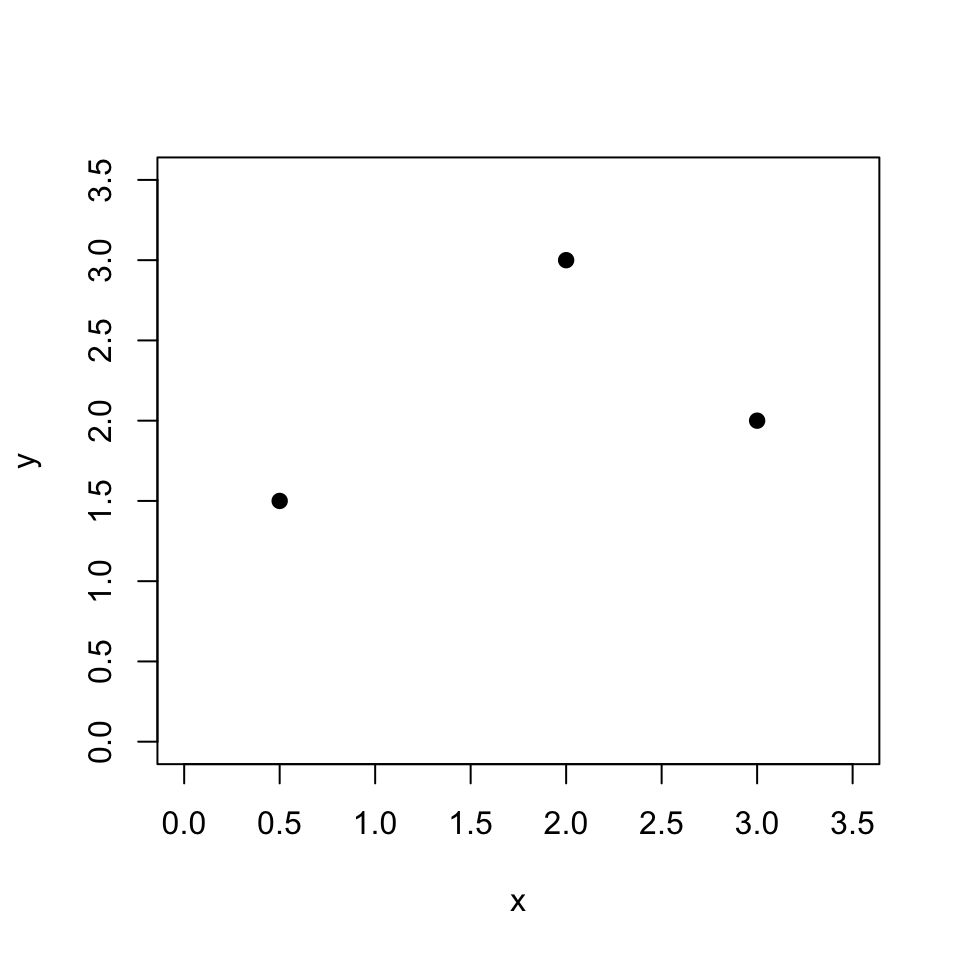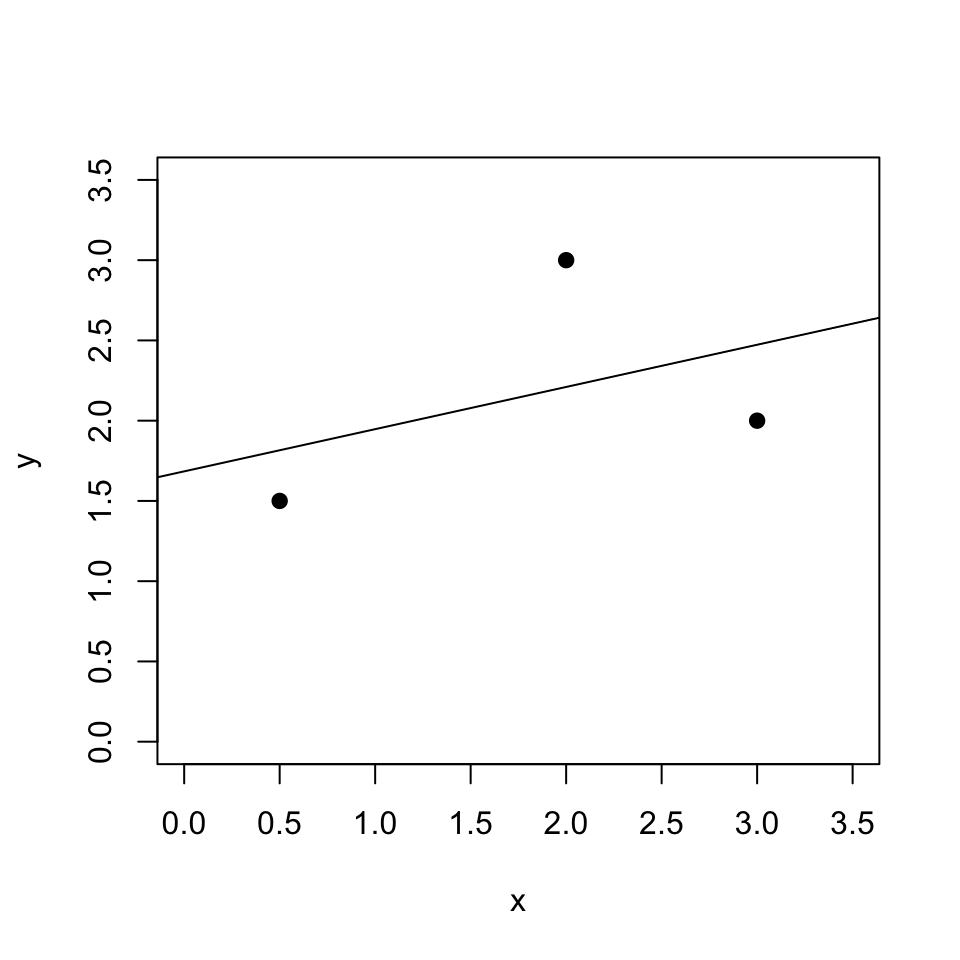## Objective

We’re going to provide a geometric interpretation of OLS regression. It shows how the fitted values from an OLS regression can be seen as the projection of the outcome data onto a plane (or hyperplane) spanned by the explanatory variables.

The simplest way to show this is with two data points and one explanatory variable (a constant). Ben Lambert does this nicely in this video.

Here I will show it in the next simplest case, where there are three data points and two explanatory variables (a constant plus one other). This requires some visualization in 3d, which we will do with the rgl package in R.

## The data

Our data consist of three units for which we observe two attributes, y and x.

y <- c(1.5,2,3)
x <- c(.5,3,2)

## The conventional geometry of regression: attributes as dimensions

Usually, we would visualize this data by assigning each attribute of the data to a dimension and representing each observation with a point:

plot(x, y, pch = 19, xlim = c(0, 3.5), ylim = c(0, 3.5))We can regress y on x and add the regression line to the figure:

the_lm <- lm(y ~ x)
plot(x, y, pch = 19, xlim = c(0, 3.5), ylim = c(0, 3.5))
abline(the_lm)OLS gives us the line that minimizes the sum of the squared residuals, i.e. vertical distances between the points and the line.

This approach to visualizing two attributes works no matter how many observations we have.

## Vector space geometry of regression: observations as dimensions

To see OLS as orthogonal projection, we need to reverse the role of attributes and observations in our geometry. Now, each observation gets a dimension and each attribute gets a data point, or more precisely a vector.

First we define a new attribute, the constant:

constant <- c(1,1,1)

Now we’ll visualize the data in 3d using the rgl package. We’ll put the plotting commands in a function for reuse:

# I write this as a function for reuse below
base_plot <- function(maxval = 3){
plot3d(x = 0, y = 0, z = 0, type = "n",
xlim = c(0,maxval),
ylim = c(0,maxval),
zlim = c(0,maxval),
xlab = "Obs 1", ylab = "Obs 2", zlab = "Obs 3")
text3d(x, texts = "x")
lines3d(x = rbind(c(0,0,0), x))
text3d(constant, texts = "Constant")
lines3d(x = rbind(c(0,0,0), constant))
text3d(y, texts = "y")
lines3d(x = rbind(c(0,0,0), y), col = "red")
}
base_plot() 

It’s hard to get used to this representation of the data because we’re so used to thinking of data points as observations and dimensions as attributes.

(Note you should be able to rotate the plot and zoom in and out!)

Now, we want to determine a linear combination of x and constant that produces an appealing vector of fitted values for y. All linear combinations of x and constant lie on a plane; this is the plane spanned by the vectors x and constant, and it is referred to as the column space of $$\mathbf{X}$$. ($$\mathbf{X}$$ in general refers to the matrix of explanatory variables; here it has two columns, x and constant.) We can represent this plane below with a grid of points:1

base_plot()
df <- data.frame(rbind(x, constant, c(0,0,0)))
colnames(df) <- c("Obs 1", "Obs 2", "Obs 3")
plane_lm <- lm(Obs 3 ~ Obs 1 + Obs 2, data = rbind(df))
points_df <- expand.grid(x = seq(0, 3, .1), y = seq(0, 3, .1))
points_df$z <- coef(plane_lm)["Obs 1"]*points_df$x + coef(plane_lm)["Obs 2"]*points_df\$y
points3d(points_df, alpha = .3) 

Note that x and constant lie on this plane, but y does not.

The fitted values from the OLS regression of y on x can themselves be plotted as a vector. This vector lies on the plane spanned by x and constant, and its tip is as close as possible to the tip of the y vector.

base_plot()
points3d(points_df, alpha = .3)
the_pred <- predict(the_lm)
points3d(x = the_pred, y = the_pred, z = the_pred, col = "blue", size = 5)
lines3d(rbind(c(0,0,0), the_pred), col = "red", lwd = .5)
lines3d(rbind(y, the_pred), col = "black", lwd = .5)

Thus by finding the coefficients that minimize the sum of squared residuals, we produce a vector of fitted values that is as close as possible to y while still residing in the column space of $$\mathbf{X}$$.

We can also construct the vector of fitted values by combining the x and constant vectors according to the regression coefficients:

base_plot()
points3d(points_df, alpha = .3)
points3d(x = the_pred, y = the_pred, z = the_pred, col = "red", size = 5)
extended_constant <- coef(the_lm)["(Intercept)"]*constant
lines3d(rbind(c(0,0,0), extended_constant), col = "orange")
lines3d(rbind(extended_constant, extended_constant +  coef(the_lm)["x"]*x), col = "blue")
lines3d(rbind(y, the_pred), col = "black", lwd = .5)

We get to the prediction vector by extending in the direction of the constant vector (the yellow line) and then in the direction of the x vector (the blue line); we could do it in the opposite order too.

## Beyond three data points

It’s impossible to visualize this beyond the case of three data points, but the intuition we get from three data points (or even two, if we’re just estimating the intercept) applies: the data $$\mathbf{y}$$ is a vector in $$n$$-multidimensional space; the explanatory variables define a hyperplane in $$n$$ dimensions; the regression yields a set of coefficients $$\hat{\beta}$$ that combine the explanatory variables to yield a prediction vector $$\hat{y}$$ that is closest to $$y$$ while still being on the hyperplane defined by the explanatory variables.

## Geometric derivation of OLS2

Let $$\mathbf{X}$$ denote the matrix of explanatory variables, including the constant. $$\mathbf{y}$$ is the vector of outcomes and $$\hat{\mathbf{y}} = \mathbf{X} \hat{\beta}$$ is the vector of predictions, with $$\hat{\beta}$$ the coefficients on the columns of $$\mathbf{X}$$.

As noted above, $$\hat{\mathbf{y}}$$ can be seen as a vector in the column space of $$\mathbf{X}$$; its tip is as close as we can get to the tip of $$\mathbf{y}$$ while still being in the column space of $$\mathbf{X}$$. This implies that the vector connecting $$\hat{\mathbf{y}}$$ and $$\mathbf{y}$$ (given by $$\hat{\mathbf{y}} - \mathbf{y}$$) is orthogonal to the column space of $$\mathbf{X}$$. This in turn implies that 3

$\mathbf{X}'(\hat{\mathbf{y}} - \mathbf{y}) = 0.$

Expanding this,

$\mathbf{X}'\mathbf{X} \hat{\beta} - \mathbf{X}'\mathbf{y} = 0$

so that

$\mathbf{X}'\mathbf{X} \hat{\beta} = \mathbf{X}'\mathbf{y}$

and

$\hat{\beta} = (\mathbf{X}'\mathbf{X})^{-1}\mathbf{X}'\mathbf{y},$

which is a remarkably simple derivation of OLS.

1. I couldn’t get planes3d to render properly with webgl.↩︎

2. This derivation comes from this video by Ben Lambert.↩︎

3. Ben Lambert states this, but I don’t see why it follows. I understand that orthogonality implies a product of zero, but I don’t see why orthogonality to the column space of $$\mathbf{X}$$ implies orthogonality to $$\mathbf{X}$$.↩︎